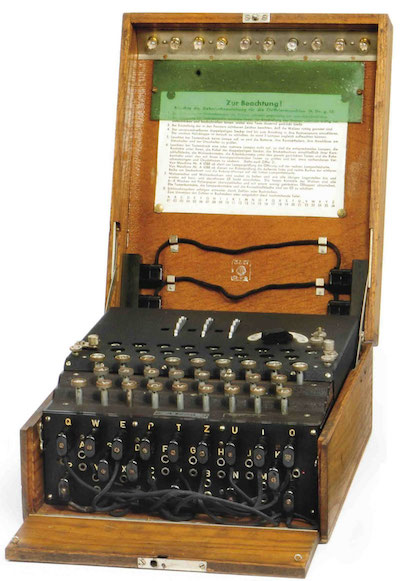An ~Enigma~ machine, famed for its inner complexity.

Recurrent Neural Networks (RNNs) are Turing-complete. In other words, they can approximate any function. As a tip of the hat to Alan Turing, let’s see if we can use them to learn the Enigma cipher.

## A Brief History of Cryptanalysis

According to Wikipedia, “Cryptanalysis is the study of analyzing information systems in order to study their hidden aspects.”

By hand. Long ago, cryptanalysis was done by hand. People would count the frequencies of symbols, compare encrypted text to decrypted text, and try to find patterns. It was a meticulous process which required days and weeks of concentration. Starting with World War II, the heavy lifting was transferred to machines and humans experts started spending their time on problems in pure mathematics which enabled them to crack all but the toughest ciphers. But even today, cryptanalysts spend much of their time meticulously dissecting the structure of the cipher they’re trying to crack. Does this need to be the case?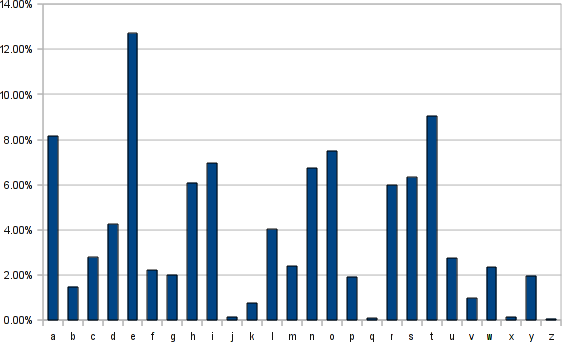The frequency table is a classic codebreaking tool

Black boxes. The Black Box theory of cryptograpy states “If the output of an algorithm when interacting with the [encrytion] protocol matches that of a simulator given some inputs, it ‘need not know’ anything more than those inputs” (Wikipedia). If that’s the case, we should be able to mimic complicated ciphers such as the Enigma without knowing anything about how they work. All we need is a way to approximate the function $$f_{Enigma}$$ which maps from plaintext to ciphertext

$\mathbf{ciphertext} = f_{Enigma}(\mathbf{key}, \mathbf{plaintext})$

Can neural nets help? In this post, I’ll do this with an RNN parameterized by weights $$\theta$$ which we’ll train using gradient descent. In other words, we’ll try

\begin{align} L_t[i,j] &= f_{Enigma}(\mathbf{key}, \mathbf{plaintext})\\ &\approx f_{RNN}(\theta, \mathbf{key}, \mathbf{plaintext})\\ \end{align}

## Deep Learning for Cryptanalysis

Framing the problem. Let’s consider the general problem of decryption where there is a 1:1 mapping between the plaintext and ciphertext. If you think of the plaintext as English and the ciphertext as a strange foriegn language, the training objective resembles that of machine translation. Given a string of letters in English - let’s use “You know nothing Jon Snow” as an example - we should learn to scramble them according to the rules of the cipher.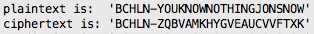Basic training objective where "BCHLN" is the key

Choose a model. Framed as a 1:1 sequence-to-sequence task, we can see that an RNN (we’ll use a Long Short Term Memory (LSTM) cell) might perform well. These models are capable of capturing complex sequential patterns where events that happened many time steps in the past can determine the next symbol.

Solve something simpler. Before tackling a really tough problem like the Enigma, it’s a good idea to solve something simpler. One of my favorite ciphers is the Vigenere cipher, which shifts the plaintext according to the letters in a keyword (see gif below). For a more in-depth description, check out the Vigenere Wikipedia page.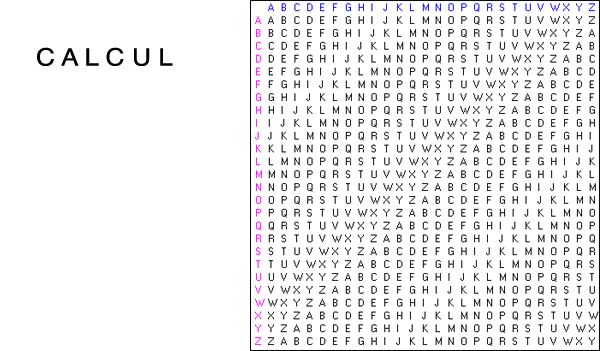Using the Vigenere cipher to encrypt plaintext "CALCUL" with keyword "MATHS" (repeated).

Results. The Vigenere cipher was easy. A mere 100,000 steps of gradient descent produced a model which learned the decryption function with 99% accuracy.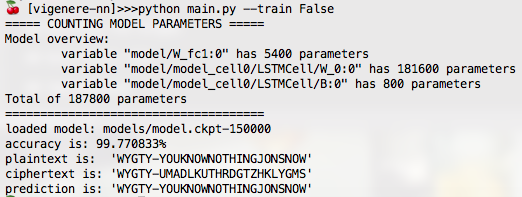A sample output from the model I trained on the Vigenere cipher.

You can find the code on my GitHub.

## Learning the Enigma

The Enigma. Now we’re ready for something a lot more complex: the Nazi Enigma. Its innards consisted of three rotating alphabet wheels, several switchboards, and ten cables. All told, the machine had 150,738,274,900,000 possible configurations!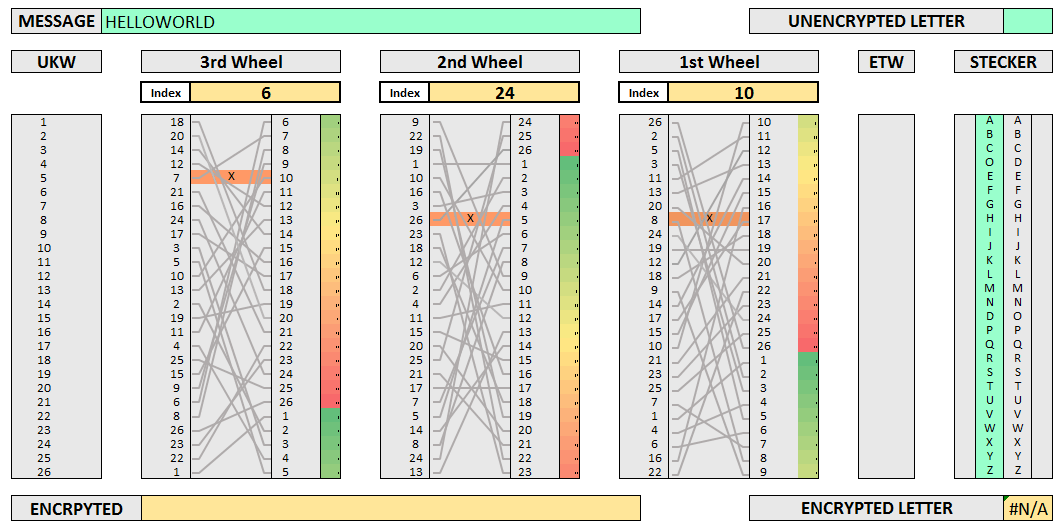How the Enigma works. Note that the three wheels can rotate as the decoding process unfolds

Background. Breaking the Enigma was an incredible feat - it even inspired the 2014 film The Imitation Game starring Benedict Cumberbatch as Alan Turing. Turing was one of the most important figures in the project. He also introduced the notion of Turing-completeness. In an ironic twist, we’ll be using a Turing-complete algorithm (the LSTM) to learn the Enigma.

We’ll train the model on only one permutation of switchboards, cables, and wheels. The keyword, then, is three letters which tell the model the initial positions of the wheels.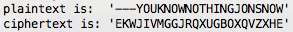Basic training objective where "EKW" is the keyword. The keyword defines the initial positions of the three alphabet wheels

Making it happen. I synthesized training data on-the-fly using the crypto-enigma Python API and checked my work on a web-based Enigma emulator. I used each training example only once to avoid the possibility of overfitting.

The model needed to be very large to capture all the Enigma’s transformations. I had success with a single-celled LSTM model with 3000 hidden units. Training involved about a million steps of batched gradient descent: after a few days on a k40 GPU, I was getting 96-97% accuracy!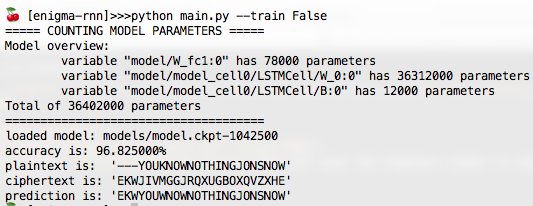A sample output from the model I trained on the Enigma cipher.

You can find the code on my GitHub.

## Modern encryption

Learning the Enigma is interesting, but these days it has no practical use. Modern encryption uses public-key factoring algorithms such as RSA. RSA is a different beast from the Enigma, but in theory we could also learn it with deep learning. In practice, this is difficult because RSA uses modulus and multiplication of large integers. These operations are difficult to approximate with RNNs. We need further algorithmic advances in deep learning like the Neural GPU or the Differential Neural Computer to make this problem even sort of feasible (and even then, this will be even close to the most efficient approach).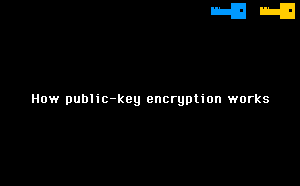Public-key encryption. In theory, we could learn the RSA with deep learning but it presents many practical difficulties

## Implications

Cryptanalysis. In this post I’ve shown that it is possible to use deep learning to learn several polyalphabetic ciphers including the Enigma. This approach is interesting because it’s very general: given any “blackbox” cipher, we can learn the function that maps the ciphertext to the plaintext. There are countless programs that can analyze only one type or class of cypher, but this is the first instance$$^{*}$$ of a cipher-agnostic cryptanalysis program powered by deep learning.

AI. In the past several years, Deep Reinforcement Learning has enabled an impressive series of breakthroughs in the field of Artificial Intelligence (AI). Many believe that these breakthroughs will enable machines to perform complex tasks such as driving cars, understanding text, and even reasoning over memory. This project suggests that AIs built from neural networks could also become effective code breakers.The original Enigma cracker (a Bombe machine). Crypto Museum

$$^{*}$$based on an Arxiv search and Google Scholar results